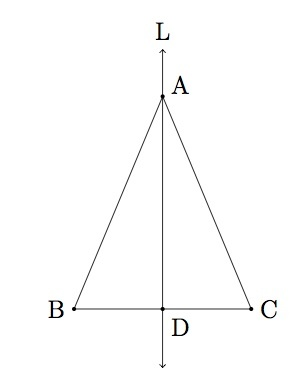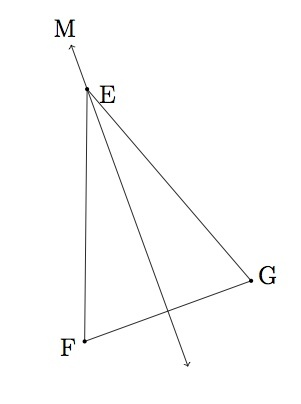# Reflections and Isosceles Triangles

Alignments to Content Standards: G-CO.B

Suppose $ABC$ is an isosceles triangle with side $\overline{AB}$ congruent to side $\overline{AC}$ as pictured below:Also pictured above is the midpoint $D$ of side $\overline{BC}$. Let $L$ denote line $\overleftrightarrow{AD}$ and let $r_L$ denote the map of the plane determined by reflection about the line $L$.

1. Show that $r_L(A) = A$, $r_L(B) = C$, and $r_L(C) = B$.
2. Using part (a) show that $r_L$ maps the triangle $ABC$ to itself.
3. Suppose $EFG$ is a triangle in the plane and that there is a line $M$ so that reflection about $M$ maps triangle $EFG$ to itself. Show that triangle $EFG$ is an isosceles triangle.
4. Using parts (b) and (c), show that a triangle is isosceles if and only if there is a reflection of the plane that preserves the triangle.

## IM Commentary

This activity is one in a series of tasks using rigid transformations of the plane to explore symmetries of classes of triangles, with this task in particular focussing on the class of isosceles triangles. Students will have previously seen similar material in earlier grades (e.g., standard 4.G.3: "Recognize a line of symmetry for a two-dimensional figure as a line across the figure such that the figure can be folded along the line into matching parts. Identify line-symmetric figures and draw lines of symmetry."), and this task is a similar exploration with a level of mathematical precision more appropriate for high schools students. In particular, the task has students link their intuitive notions of symmetries of a triangle with statements proving that said triangle is unmoved by applying certain rigid transformations.

The mechanism through which students do this is via congruence proofs, so the task illustrates the general relationship between congruence and rigid transformations, as fits naturally under the cluster "understand congruence in terms of rigid motions." At the high school level, students will need to use the definition of reflections and the teacher may wish to recall this before having students work on the problem. The instructor may wish to discuss with students how to draw an appropriate picture for part (b) and part (c) as this will be essential in order to make progress on these problems. In addition, it may be necessary to recall the definition of reflection about a line $L$: reflection about $L$ maps each point on $L$ to itself and if $P$ is not on $L$ then reflection about $L$ maps $P$ to $Q$ when $L$ is the perpendicular bisector of segment $PQ$ as pictured below:This task is mainly intended for instructional purposes: for students with a strong visual sense of geometry, rigid motions of the plane provide a rich source of ideas which can be used to grasp many important geometric ideas.

## Solution

1. Since the reflection over line $L$ leaves each point on $L$ fixed, we have $r_L(A) = A$ since $A$ lies on $L$. Since $B$ and $C$ do not lie on $L$, in order to show that $r_L(B) = C$ and $r_L(C) = B$ we need to show that $L$ is the perpendicular bisector of segment $\overline{BC}$.

Note that we are given that segment $\overline{AB}$ is congruent to segment $\overline{AC}$. Since $D$ is the midpoint of segment $\overline{BC}$ this means that segment $\overline{BD}$ is congruent to segment $\overline{CD}$. Finally segment $\overline{DA}$ is congruent to segment $\overline{DA}$. So this means, by SSS, that triangle $ABD$ is congruent to triangle $ACD$. Since angles $BDA$ and $CDA$ together add up to 180 degrees and they are congruent it follows that they are both right angles. Therefore $L$ is perpendicular to segment $\overline{AB}$ and it is the bisector of $\overline{AB}$ by assumption.

2. From part (a) we know that $r_L(A) = A$, $r_L(B) = C$, and $r_L(C) = B$. Reflections map line segments to line segments so it follows that $r_L$ maps side $\overline{AB}$ to side $\overline{AC}$, side $\overline{AC}$ to side $\overline{AB}$ and side $BC$ to itself. Hence $r_L$ maps triangle $ABC$ to itself. Note that although $r_L$ maps side $\overline{BC}$ of triangle $ABC$ to itself, the map interchanges vertices $B$ and $C$ so the orientation of this segment is reversed.

3. We are given that $r_M$ maps triangle $EFG$ to itself. Suppose we look at $r_M(E)$. Since $r_M$ preserves triangle $EFG$ it must interchange the vertices of $E, F, G$ in some way. One possibility would be that it leaves them all alone, that is $E$ maps to $E$, $F$ maps to $F$, and $G$ maps to $G$. This can not happen, however, because a reflection of the plane can not leave $\triangle EFG$ in its original position. If two of the vertices are preserved by $r_M$ then the third must be also as there is no freedom to send it to either of the two fixed vertices. So this means that the reflection $r_M$ must leave exactly one vertex of $\triangle EFG$ fixed and it must exchange the other two vertices. For simplicity, let's assume that $r_M(E) = E$, $r_M(F) = G$, and $r_M(G) = F$. This situation is pictured below:Since $r_M$ preserves distances this means that $|EF| = |r_M(EG)| = |EG|$ and so $\triangle EFG$ is isosceles.

4. Part (b) shows that for each isosceles triangle $ABC$ there is at least one line $L$ so that $r_L$ maps triangle $ABC$ to itself. Part (c) shows that if there is a line $L$ so that $r_L$ maps triangle $ABC$ to itself then triangle $ABC$ is isosceles. Putting these two statements together we see that triangle $ABC$ is isosceles if and only if there is a line $L$ so that $r_L$ maps triangle $ABC$ to itself.